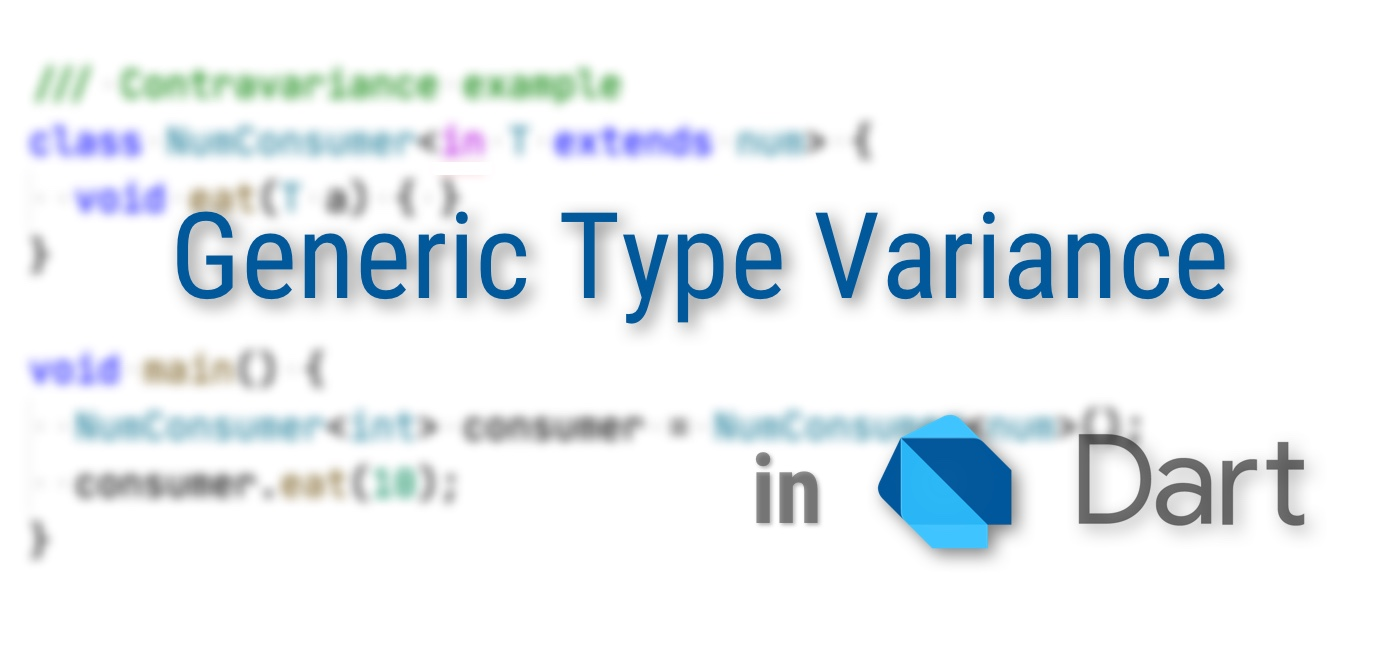# Generic Type Variance in Dart

Yingxin Wu · 2020-02-14Dart支持泛型编程（generics）特性，带来便利的同时也带来了相应的复杂度。比如应该如何判定两个泛型类型之间父/子类型关系？例如，以下语句通常会被认为是合理的：

``````List<int> integers = <int>[1, 2];
List<num> numbers = integers;
``````

## 变体的种类

1. Covariance
co-” 词根一般有「共同、协同」的含义，意味着「同质、和谐」，因此这就是最直观的一种变体，例如：`Iterable<num> numbers = <int>[1, 2]`。即，如果类型参数具有子类型关系，就认为这两个泛型类型也具有子类型关系
2. Contravariance
contra-” 词根具有「相反」的含义, 因此它和covariance的赋值方向正好相反：`Writer<int> intWriter = Writer<Object>()`
3. Invariance
in-” 表示「否定」，这里表示不接受变体，只有类型严格相同，变量才可以相互赋值

## Producer / Consumer

### 生产者

``````class Producer<out T> {
T get next => …;
}

Producer<num> numbers = Producer<int>();
print(numbers.next);
``````

`Producer<int>`生产`int`，而`int``num`的一种，因此把它理解为「`num`的生产者」是自然且安全的。

Covariance约束之下，类型参数`T`将不能用于任何具有输入特征的地方：

``````class Producer<out T> {
void add(T a) { … }
^ The 'out' type parameter 'T' can't be used in an 'in' position.
}
``````

``````Iterable<num> numbers = <int>[1, 2];
numbers = numbers.map((x) => x * 2);
``````

### 消费者

``````class Consumer<in T> {
void eat(T a) { … }
}
``````

``````final consumer = Consumer<num>();
consumer.eat(1.0);
consumer.eat(2);
``````

``````final Consumer<int> consumer = Consumer<num>();
consumer.eat(10);
``````

`Consumer<num>`接受`num`类型的输入，当然也能接受`int`类型的输入，所以使用一个`Consumer<int>`类型的变量来引用它是安全的。这样，编译器就能帮助我们避免输入不希望的数据类型，如`double`

``````class Consumer<in T> {
final T first;
^ The 'in' type parameter 'T' can't be used in an 'out' position.
}
``````

### 生产者 + 消费者

``````// Dart目前的默认模式，没有约束
void write(T a) {}
}
``````

``````// 1. covariance
nrw.write(0.0);
``````

``````// 2. contravariance
``````

``````class ReadWrite<inout T> {
void write(T a) {}
}
``````

``````ReadWrite<int> irw = ReadWrite<int>();
irw.write(10);

// 如果希望适用面更广，就需要使用父类了
..write(11)
..write(2.0);
``````

## Use-site Variance

``````void pipeline(ReadWrite<num> from, ReadWrite<num> to) {
}

// 不能这样做
^ The argument type 'ReadWrite<int>' can't be assigned to the parameter type 'ReadWrite<num>'.
``````

`int`类型的数据作为`num`类型取出然后写入，这个合理的要求也被拒绝了。

``````void pipeline(ReadWrite<out num> from, ReadWrite<num> to) {
}

// 允许
``````

``````void pipeline<T extends num>(ReadWrite<T> from, ReadWrite<num> to) {
}
``````

## 现状

Dart的declaration-site variance特性目前处于实验阶段，为了体验这个特性，编译/运行时需要添加参数：`--enable-experiment=variance`Analyzer则可以在`analysis_options.yaml`文件中配置：

``````analyzer:
enable-experiment:
- variance
``````

use-site variance特性的GitHub issue尚未被纳入任何Project，估计还需要等待比较长的一段时间才能够得到引入吧。